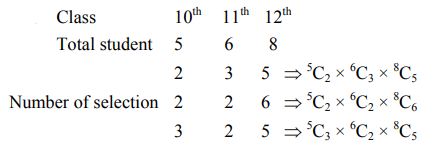# There are 5 students in class 10,6 students in class 11 and 8`
Question:

There are 5 students in class 10,6 students in class 11 and 8 students in class 12 . If the number of ways, in which 10 students can be selected from them so as to include at least 2 students from each class and at most 5 students from the total 11 students of class 10 and 11 is $100 \mathrm{k}$, then $\mathrm{k}$ is equal to_______.

Solution:'

$\Rightarrow$ Total number of ways $=23800$

According to question

$100 \mathrm{~K}=23800$

$\Rightarrow \mathrm{K}=238$